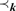Hostname: page-component-5db6c4db9b-mcx2m Total loading time: 0 Render date: 2023-03-23T15:50:38.775Z Has data issue: true Feature Flags: { "useRatesEcommerce": false } hasContentIssue true

# ALMOST GALOIS ω-STABLE CLASSES

Published online by Cambridge University Press:  22 July 2015

## Abstract

Theorem. Suppose that k = (K,$$\prec_k$$) is an 0-presentable abstract elementary class with Löwenheim–Skolem number 0, satisfying the joint embedding and amalgamation properties in 0. If K has only countably many models in 1, then all are small. If, in addition, k is almost Galois ω-stable then k is Galois ω-stable. Suppose that k = (K,$$\prec_k$$) is an 0-presented almost Galois ω-stable AEC satisfying amalgamation for countable models, and having a model of cardinality 1. The assertion that K is 1-categorical is then absolute.

## Keywords

Type
Articles
Information
The Journal of Symbolic Logic , September 2015 , pp. 763 - 784

## Access options

Get access to the full version of this content by using one of the access options below. (Log in options will check for institutional or personal access. Content may require purchase if you do not have access.)

## References

#### REFERENCES

Baldwin, John T., Categoricity, University Lecture Notes, vol. 51, American Mathematical Society, Providence, RI, 2009, www.math.uic.edu/∼jbaldwin.
Baldwin, John T., Amalgamation, absoluteness, and categoricity, Proceedings of the 11th Asian Logic Conference, 2009 (Arai, , Feng, , Kim, , Wu, , and Yang, , editors), pp. 2250, World Scientific Publisher, Hackensack, NJ, 2012.Google Scholar
Baldwin, J. T., Friedman, Sy, Koerwien, M., and Laskowski, C., Three red herrings around vaught’s conjecture, Transactions of the American Mathematical Society, to appear.
Baldwin, J. T., Hyttinen, T., and Kesälä, M., Beyond first order logic: From number of structures to structure of numbers part II. Bulletin of Iranian Mathematical Society, vol. 39 (2013), no. 1, pp. 2748, available online at http://www.iranjournals.ir/ims/bulletin/.Google Scholar
Baldwin, J. T. and Larson, Paul, Iterated elementary embeddings and the model theory of infinitary logic, preprint, http://homepages.math.uic.edu/∼jbaldwin/pub/.
Boney, W., Computing the number of types of infinite length, preprint.
Enayat, A., On certain elementary extensions of models of set theory. Transactions of the American Mathematical Society, vol. 283 (1984), pp. 705715.CrossRefGoogle Scholar
Gao, Su, Invariant Descriptive Set Theory, CRC Press, Boca Raton, FL, 2009.Google Scholar
Harnik, V. and Makkai, M., A tree argument in infinitary model theory. Proceeedings of the American Mathematical Society, vol. 67 (1977), pp. 309314.CrossRefGoogle Scholar
Hutchinson, John E., Elementary extensions of countable models of set theory, this Journal, vol. 41 (1976), pp. 139145.Google Scholar
Hyttinen, T. and Kesälä, M., Independence in finitary abstract elementary classes. Annals of Pure and Applied Logic, vol. 143 (2006), no. 13, pp. 103138.CrossRefGoogle Scholar
Jarden, A. and Shelah, S., Non-forking frames in abstract elementary classes. Annals Pure and Applied Logic, vol. 164 (2013), pp. 135191.CrossRefGoogle Scholar
Keisler, H. J., Model theory for Infinitary Logic, North-Holland, Amsterdam, 1971.Google Scholar
Keisler, H. J. and Morley, M., Elementary extensions of models of set theory. Israel Journal of Mathematics, vol. 5 (1968), pp. 331348.Google Scholar
Knight, J. F., Montalban, A., and Schweber, N., Computable structures in generic extensions, preprint, 2014.
Kueker, D. W., Abstract elementary classes and infinitary logics. Annals of Pure and Applied Logic, vol. 156 (2008), pp. 274286.CrossRefGoogle Scholar
Larson, Paul, Scott processes, preprint.
Marker, D., Scott ranks of counterexamples to Vaught’s conjecture, Notes from 2011, http://homepages.math.uic.edu/∼marker/harrington-vaught.pdf.
Marker, D., Model theory and infinitary logic, Notes from 2013, http://homepages.math.uic.edu/∼marker/math512-F13/.